## Introduction

Demographic history includes when and where a particular population come from, its genetic relationship to each other populations, and how its population size changes over time.

The POPdemog package produces plots which visualize population demographic history from the description of the demographic model used as input to population genetic simulator programs, such as ms , msHot , MaCS , and Cosi . Msprime's simulation scripts  are also supported by this package since they can be translated into ms-compatible commands. We plan to support additional simulators in the future. Please check the list of supported simulators for updates.

The POPdemog package provides three easy-to-use functions: PlotMS(), PlotMMig(), and PlotMig()PlotMS() is the main function. It converts the demographic information from a simulation script or simulation parameter file into a plot of phylogenetic tree with arrows showing migrations between lineages.  The PlotMMig() and PlotMig() functions are designed to deal with complex migration events.  PlotMMig() gives an overview of migrations over time, and PlotMig() shows migrations at a specified time point in fine detail. In addition, there is a function NRuler() which adds a population size ruler to the plot.

This tutorial assumes that the user has some experience with a population genetics simulator program and some experience generating plots using R. An understanding of effective population size is also helpful. We will use a demographic model from Cosi to explore the power of POPdemog to visualize population history. This tutorial also includes many additional examples which show how to use the POPdemog package with different models and different simulator programs.

In this tutorial, function names are italicized [function()], and parameters and code is presented in mono-spaced font [option & code].

Note: Currently, this POPdemog package does not check the simulation program input for correctness, but assumes the simulation program input has been validated by the simulation program.

[top]

## Supported Simulators

Simulator Keyword Notes
Cosi2 “cosi” Use parameter file as input
ms “ms”
msHot “mshot”
msprime “msprime” Must first convert to ms-compatible input format
MaCS “macs”
SCRM “scrm”

[top]

## Installing the POPdemog package

We are working on submitting this package to the CRAN. User can also download and install it with the R command:

install.package("POPdemog_1.0.3.tar.gz", repos=NULL, source=TRUE)


For R version >= 3.3.0, package POPdemog can be installed from the source file

install.packages("https://github.com/YingZhou001/POPdemog/raw/master/POPdemog_1.0.3.tar.gz", repos=NULL)


library(POPdemog)


[top]

## Plotting demographic history with PlotMS()

The PlotMS() function requires the input.file or input.cmd, and the type of the input to be specified.

###Script input: input.file, input.cmd, and type

The input.file contains all the demographic history information, it can be a copy of the simulation command line or the parameter file.

For example, the ms command line stored in a text file can be the input.file

./ms 1 1 -r 25 250001 -t 2.5 -I 4 50 50 50 60 -n 1 10 -n 2 10 -n 3 10 -n 4 10 -em 0 1 4 0.32 -em 0 4 1 0.32 -em 0 3 4 0.08 -em 0 4 3 0.08 -em 5e-04 2 1 2000 -em 6e-04 2 1 0 -ej 7e-04 2 4 -en 0.02 4 2.4 -en 0.035 1 0.77 -en 0.04 3 0.77 -en 0.1997 4 0.0125 -en 0.1998 3 0.00149253731343284 -en 0.1999 1 0.005 -ej 0.2 3 1 -em 0.1996 1 4 0 -em 0.1995 4 1 0 -em 0.1994 3 4 0 -em 0.1993 4 3 0 -en 0.3499 1 0.00117647058823529 -ej 0.35 1 4 -en 1.7 4 1.25


and the parameter file (from the software Cosi2) of Cosi can be the input.file.

# sample file
# comments have #s in front of them
# newlines don't matter.

#-- options that could be uncommented
#infinite_sites yes
#random_seed 12345   # Specifies a particular random number seed

# in bp.
length 250000

# per bp per generation
mutation_rate 1.5e-8

recomb_file model.out
gene_conversion_relative_rate 0.3

# population info

pop_define 1 european
pop_define 3 african-american
pop_define 4 asian
pop_define 5 african

#european
pop_size 1 100000
sample_size 1 50

#african american
pop_size 3 100000
sample_size 3 50

#asian
pop_size 4 100000
sample_size 4 50

#african
pop_size 5 100000
sample_size 5 60

pop_event migration_rate "afr->eur migration" 5 1 0. .000032
pop_event migration_rate "eur->afr migration" 1 5 0 .000032
pop_event migration_rate "afr->as migration" 5 4 0. .000008
pop_event migration_rate "as->afr migration" 4 5 0 .000008
pop_event split "african to aa" 5 3 7.0

pop_event change_size "agriculture - african" 5 200 24000
pop_event change_size "agriculture - european" 1 350 7700
pop_event change_size "agriculture - asian" 4 400 7700
pop_event bottleneck "african bottleneck" 5 1997 .008
pop_event bottleneck "asian bottleneck" 4 1998 .067
pop_event bottleneck "european bottleneck" 1 1999 .02

pop_event split "asian and european split" 1 4 2000
pop_event migration_rate "afr->eur migration" 5 1 1996 0
pop_event migration_rate "eur->afr migration" 1 5 1995 0
pop_event migration_rate "afr->as migration" 5 4 1994 0
pop_event migration_rate "as->afr migration" 4 5 1993 0

pop_event bottleneck "OoA bottleneck" 1 3499 .085
pop_event split "out of Africa" 5 1 3500

pop_event change_size "african pop size" 5 17000 12500


We will store the preceding ms command line in the file “sample.ms.cmd”, and use it in examples later in this tutorial.

We also provide the string input option input.cmd, which allows the user to pass the simulation command line as a string to the PlotMS() function. The type parameter must be specified to so that PlotMS() can interpret the input correctly.

[top]

###Scale population size: N4 Effective population size is an important parameter in simulations with ms-like script (msHot, scrm, and MaCS). It is used to scale the population size and the time for demographic events. The N4 parameter has the same meaning of the $$4N0$$ parameter in ms, which must be specified in order to give the correct times for demographic events and the accurate sizes for populations.

The N4 parameter is not used in two situations:
1. when producing a topology plot with size.scale="topology";
2. when the simulation script do not re-scale the population size and time, such as is the case with Cosi.

[top]

###Output the demographic history

Now we can generate our first plot of demographic history from the ms file “sample.ms.cmd”, with N4 set to 10000 in this case.

PlotMS(input.file="sample.ms.cmd", type="ms", N4=10000)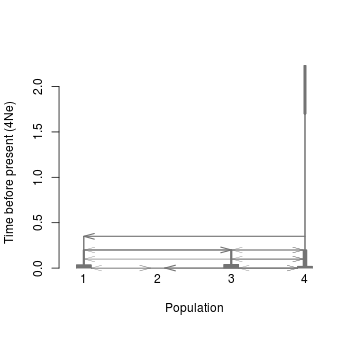This basic plot is usually not satisfactory. However, there are many options which will allow us to customize the output figure.

1.Adjust the lineage width with the size.scale parameter. Lineage width represents the population size. Here we use a logarithm function with a base of 50 to resale the population size $$N$$ so that the width of the lineage is $$log_{50}(N/N4+1)$$ in the plot.

size.scale="log", log.base =50


2.Adjust the population position with the inpos parameter (inpos[i] is the position of the ith population).

inpos=c(3,6,1,9)


3.Add color to each lineage with the col.pop parameter (col.pop[i] is the color for the $i$th population). The color of arrow is the same as the source population by default, or can be reset with the col.arrow parameter.

col.pop=c("blue", "coral3", "gold3", "brown")


4.Add population labels to each lineage with the pops parameter (pops[i] is the name for the ith population).

pops=c("European", "African American", "Asian", "African")


Adding these settings to PlotMS(), we have:

PlotMS(input.file="sample.ms.cmd", type="ms", N4=10000, size.scale="log",
log.base =50, inpos=c(3,6,1,9),
col.pop=c("blue", "coral3", "gold3", "brown"),
pops=c("European", "African American", "Asian", "African"))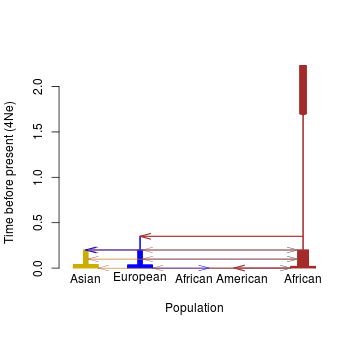If the recent events are more interesting than ancient ones, we can zoom in with

time.scale="log10year",


which will scale the y-axis as $$log_{10}(years\ before\ present)$$, using default 25 years per generation. The years per generation can be defined with the gen parameter. We can also show only the topology structure with

size.scale="topology"


which tells the function to ignore the time and population size but still display the order of demographic events. We can also adjust the font size, axis label size, and the arrow size.

cex.lab=0.6
cex.axis=0.6
length.arrowtip=0.05


If there are too many background migrations, these events can be masked by

m.adjust=0.05 #mask the migration with per generation rate less than 0.05


par(mfrow=c(1,2), las=1)
PlotMS(input.file="sample.ms.cmd", type="ms", N4=10000,
size.scale="log", log.base =50, inpos=c(3,6,1,9),
col.pop=c("blue", "coral3", "gold3", "brown"),
pops=c("European", "African American", "Asian", "African"),
title("Zoom in recent events", cex=0.8)
NRuler("topleft", Nsize=c(1e3, 1e5), Nlab=c("1e3","1e5"), N4=10000, size.scale="log",log.base=50, lwd=1, cex=0.6)
PlotMS(input.file="sample.ms.cmd", type="ms", N4=10000,
size.scale="topology", inpos=c(3,6,1,9),
col.pop=c("blue", "coral3", "gold3", "brown"),
pops=c("European", "African American", "Asian", "African"),
cex.lab=0.6, cex.axis=0.6, length.arrowtip=0.05, ylab="Time before present", m.adjust=0.05)
title("Topology plot", cex=0.8)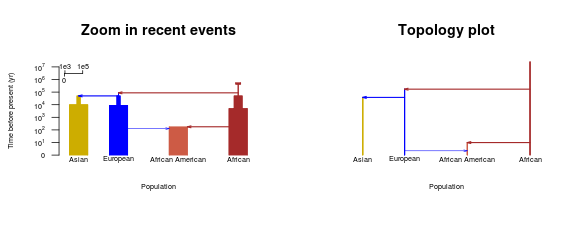[top]

## Plotting migrations

When there are multiple migrations at the particular time, arrows in the PlotMS() output might cross with the population lineages and overlap with each other. The migration events will be difficult to see in this setting, so we use function PlotMig() to output the migrations at a specified time in separate plots. We also have the PlotMMig() function to display an overview of all the migrations among simulated populations. Both of these two migration plot functions are based on the output of function PlotMS() with plot.out=F and demo.out=T.

[top]

###Using PlotMMig() to create an overview of migrations PlotMMig() will automatically output the direction and the duration of each migration. Plot settings are passed from function PlotMS() to PlotMMig() through the mig_par element of the list returned by PlotMS(). More information is available from the help(PlotMS) command in R.

#output the demographic information from PlotMS
out<-PlotMS(input.file="sample.ms.cmd", type="ms", N4=10000, size.scale="log",
log.base =50, col.arrow="black", time.scale="kyear",
col.pop=c("blue", "coral3", "gold3", "brown"),
pops=c("European", "African American", "Asian", "African"),
plot.out=F, demo.out=T,
cex.lab=0.5, cex.axis=0.6, length.arrowtip=0.05)
#plot the overview of all migrations
PlotMMig(demograph_out=out$demograph_out, mig_par=out$mig_par)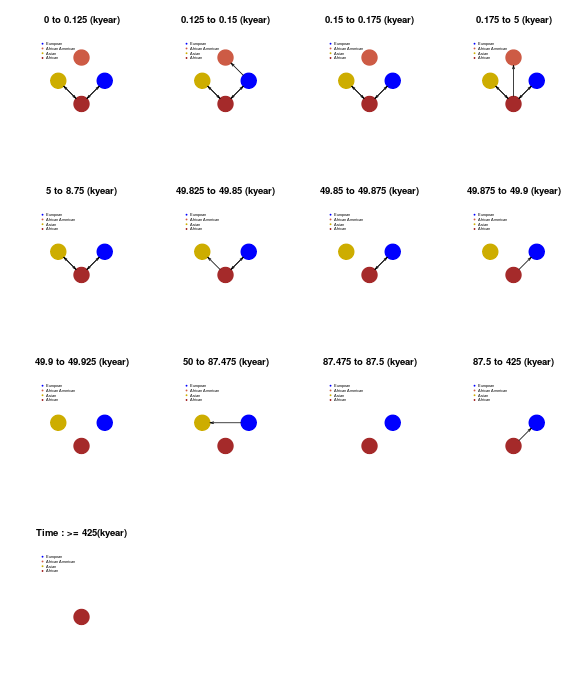[top]

###Using PlotMig() to create a fine-scale migration plot PlotMig() shows migration at a specified time. It has three options for the size.scale parameter to scale the size of the circle according to the population size at the particular time. We can ignore the size differences by setting size.scale="topology" and using toposize.scale to customize the circle sizes on the plot. We can also set size.scale="log" and size.scale="linear" to scale the circle size according to the population size. The "linear" option will give circle sizes proportional to the population sizes, while the "log" option tends to reduce the size differences and generates circles of similar sizes. More discussion on circle sizes will be found in the section Customizing the circle sizes in migration plots.

In our next example, we plot all migrations 125 years ago (with time_pt=125 and time.scale="year"). The time point(time_pt) units are specified by the time.scale parameter.

out<-PlotMS(input.file="sample.ms.cmd", type="ms", N4=10000, plot.out=F, demo.out=T)
PlotMig(time_pt=125, demograph_out=out$demograph_out, mig_par=out$mig_par,
size.scale="log", time.scale="year", log.base=20,
col.pop=c("blue", "coral3", "gold3", "brown"),
xlim=c(-8, 8), ylim=c(-8, 12));
N<-NOut(time_pt=125, demograph_out=out$demograph_out, time.scale="year"); legend("topleft", pch=20, col=c("blue", "coral3", "gold3", "brown"), bty="n", legend=paste(c("European", "African American", "Asian", "African"), " : ", N/1000, "k", sep=""), ) title(paste("Time: 125 years ago"))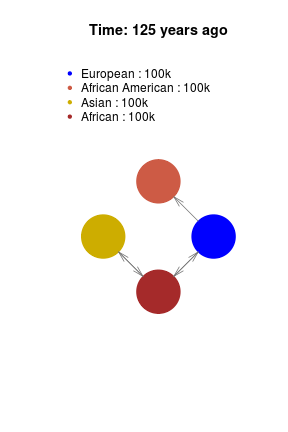In the Figure 5, the color and size of arrows can also be customized by the variables col.arrow and the length.arrowtip, please check the arguments with help(PlotMig) for more information. [top] ###Adding migrations to maps Next, we are going to see an example of adding migration plot to a map. In this example, we need to install the maps package install.package("maps")  and then load the map and save the latitude and longitude for each population in a matrix which we will call inp.map.pos. We also need to set add=T to let PlotMS() add the plot to the backgrounds. We set m.adjust=0.01 to avoid minor migrations and set toposize.scale=10 to make each population circle large enough to be easily seen. library(maps) ##initiate the world map map('legacy_world', fill=T, col="gray45", bg="lightgray", lty=0) axis(1);axis(2); ##the positions for the four populations ##European 50, 20 ##Asian 36, 112 ##African 7, 23 ##African American 38, -100 inp.map.pos<-cbind(c(20, -100, 112, 23), c(50, 38, 36, 7)) out<-PlotMS(input.file="sample.ms.cmd", type="ms", N4=10000, plot.out=F, demo.out=T) unlink("sample.ms.cmd") times<-out$mig_par$time #time points for all events times #output times points for all demographic events  ##  0.0000 0.0005 0.0006 0.0007 0.0200 0.0350 0.0400 0.1993 0.1994 0.1995 ##  0.1996 0.1997 0.1998 0.1999 0.2000 0.3499 0.3500 1.7000  for(i in times){ PlotMig(time_pt=i, demograph_out=out$demograph_out, mig_par=out$mig_par, size.scale="topology", col.pop=c("blue", "coral3", "gold3", "brown"), toposize.scale=50, add=T, map.pos=inp.map.pos, length.arrowtip=0.1, m.adjust=0.01, col.arrow=c("blue", "coral3", "gold3", "brown")); }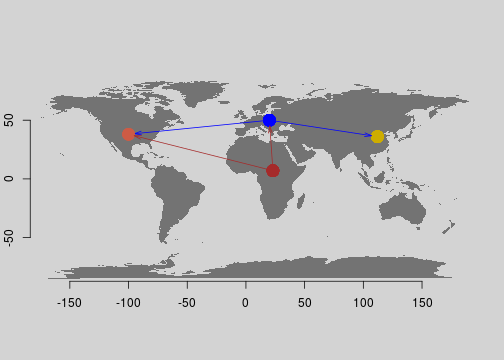[top] ## Displaying population sizes NRuler() and NOut() are two functions to display population size. NRuler() adds a population size ruler to the plot, and users can customize the ruler position, ticks on the ruler, labels on the ticks, and other figure options. The NOut() function outputs the population size numbers. It can be used with the legend() function to annotate the plot with population sizes. See the example in the section Modified Tennessen model with Neanderthal introgression for how use these two function. Generally speaking, NRuler() is for the plots generated by PlotMS(), while NOut() is for the plots generated by PlotMig(). ##More examples ### Modified Tennessen model with Neanderthal introgression cat("macs 2025 15000000 -i 10 -r 3.0e-04 -t 0.00069 -T -I 4 10 1006 1008 1 0 -n 4 0.205 -n 1 58.00274 -n 2 70.041 -n 3 187.55 -eg 0.9e-10 1 482.46 -eg 1.0e-10 2 570.18 -eg 1.1e-10 3 720.23 -em 1.2e-10 1 2 0.731 -em 1.3e-10 2 1 0.731 -em 1.4e-10 3 1 0.2281 -em 1.5e-10 1 3 0.2281 -em 1.6e-10 2 3 0.9094 -em 1.7e-10 3 2 0.9094 -eg 0.007 1 0 -en 0.007001 1 1.98 -eg 0.007002 2 89.7668 -eg 0.007003 3 113.3896 -eG 0.031456 0 -en 0.031457 2 0.1412 -en 0.031458 3 0.07579 -eM 0.031459 0 -ej 0.03146 3 2 -en 0.0314601 2 0.2546 -em 0.0314602 2 1 4.386 -em 0.0314603 1 2 4.386 -eM 0.0697669 0 -ej 0.069767 2 1 -en 0.0697671 1 1.98 -en 0.2025 1 1 -ej 0.9575923 4 1 -em 0.06765 2 4 32 -em 0.06840 2 4 0", file="model-Tennessen.cmd") #plot the demographic graph par(mfrow=c(1,2), las=1) PlotMS(input.file="model-Tennessen.cmd", type="macs", N4=30000, size.scale="log", log.base=50, inpos=c(1,4,7,9), time.scale="log10year", col.pop=c("brown", "blue", "gold3", "forestgreen"), pops=c("AFR", "EUR", "ASIA", "ARC"), cex.lab=1, cex.axis=1, xlab="", length.arrowtip=0.1) ##add population size ruler to the plot to reflect the real population sizes NRuler("topleft", Nsize=c(1e5,1e6,1e7), Nlab=c("1e5","1e6", "1e7"), N4=30000, size.scale="log",log.base=50, lwd=1, cex=0.6) title("Demographic history") PlotMS(input.file="model-Tennessen.cmd", type="macs", N4=30000, time.scale="log10year", plot.out=F, demo.out=T)->out; #log10(1000)=3 PlotMig(time_pt=1000, time.scale="year", demograph_out=out$demograph_out, mig_par=out$mig_par, col.pop=c("brown", "blue", "gold3", "forestgreen"), size.scale="linear", linear.scale=0.000003, xlim=c(0,20), ylim=c(0,30), length.arrowtip=0.08) ##annotate the real population sizes N<-NOut(time_pt=1000, time.scale="year", demograph_out=out$demograph_out)
legend("topleft",
legend=paste(c("AFR", "EUR", "ASIA", "ARC"), "size:", N),
col=c("brown", "blue", "gold3", "forestgreen"), pch=20, bty="n")
title("Migrations 1000 years ago");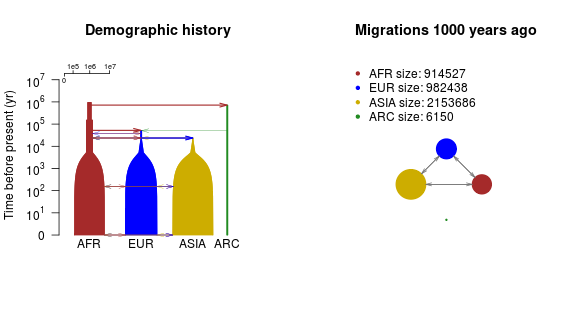[top]

### Customizing the circle sizes in migration plots

The circle size in the PlotMig() plot can be customized by the size.scale parameter. When using size.scale="log", circle size is a logarithmic function of the population size, which can be customized by the log.base parameter; when using size.scale="topology", all populations will be represented by circles of the same size, which can be scaled by the toposize.scale parameter.

par(mfrow=c(2,2))
PlotMig(time_pt=3, demograph_out=out$demograph_out, mig_par=out$mig_par,
col.pop=c("brown", "blue", "gold3", "forestgreen"),
size.scale="log", log.base=100, length.arrowtip=0.1,
xlim=c(0,15), ylim=c(0,15))
title("size.scale=\"log\", log.base=100")
PlotMig(time_pt=3, demograph_out=out$demograph_out, mig_par=out$mig_par,
col.pop=c("brown", "blue", "gold3", "forestgreen"),
size.scale="linear", linear.scale=0.000003, length.arrowtip=0.1,
xlim=c(0,15), ylim=c(0,15))
title("size.scale=\"linear\", linear.base=0.000003")
PlotMig(time_pt=3, demograph_out=out$demograph_out, mig_par=out$mig_par,
col.pop=c("brown", "blue", "gold3", "forestgreen"),
size.scale="topology", toposize.scale=0.5, length.arrowtip=0.1);
title("size.scale=\"topology\", toposize.scale=1")
PlotMig(time_pt=3, demograph_out=out$demograph_out, mig_par=out$mig_par,
col.pop=c("brown", "blue", "gold3", "forestgreen"),
size.scale="topology", toposize.scale=2, length.arrowtip=0.1);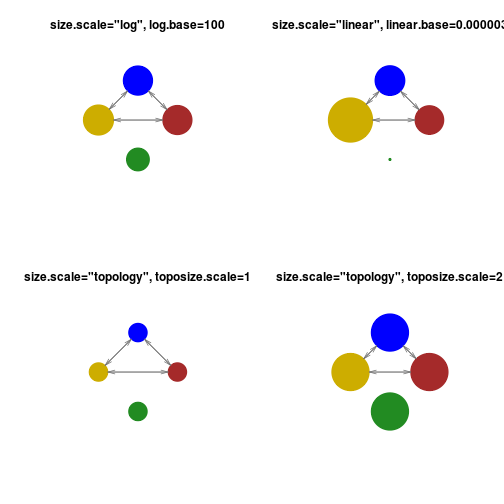[top]

###Archaic introgression model 

cat("./ms 44 1 -r 20000 50000000 -t 30000 -I 6 20 20 1 1 1 1 -en 0 1 1
-en 0 2 1 -en 0 3 1e-10 -en 0 4 1e-10 -en 0 5 1e-10 -en 0 6 1e-10
-es 0.0125 2 0.97 -en 0.02500025 7 0.25 -en 0.02500025 2 1 -ej 0.05 4 3
-ej 0.05 6 5 -en 0.05000025 3 0.25 -en 0.05000025 5 0.25 -ej 0.0500025 5 3
-en 0.050005 3 0.25 -ej 0.075 2 1 -en 0.0750025 1 1 -ej 0.1 7 3
-en 0.1000025 3 0.25 -ej 0.3 3 1 -en 0.3000025 1 1", file="test.1.ms.cmd")
PlotMS(input.file="test.1.ms.cmd", type="ms", N4=10000,
time.scale="kyear", length.arrowtip=0.1, inpos=c(1,2,5,4.5,5.5,6,3),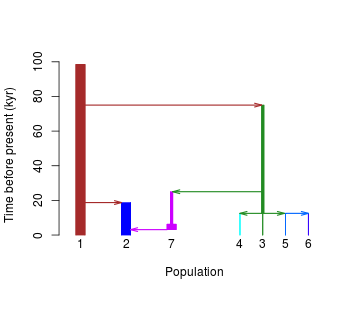[top]

###Migration model from ms

cat("./ms 15 100 -t 3.0 -I 6 0 7 0 0 8 0 -m 1 2 2.5 -m 2 1 2.5 -m 2 3 2.5
-m 3 2 2.5 -m 4 5 2.5 -m 5 4 2.5 -m 5 6 2.5 -m 6 5 2.5 -em 2.0 3 4 2.5
-em 2.0 4 3 2.5", file="test.2.ms.cmd")
PlotMS(input.file="test.2.ms.cmd", type="ms", N4=10000, col.pop="gray",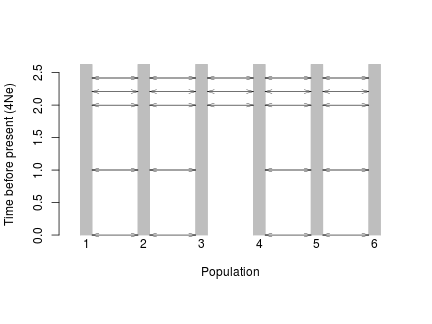[top]

###Ryan2009 model

cat("./ms 1 1 -t 1.0 -I 3 10 10 10 -n 1 1.682020 -n 2 3.736830 -n 3 7.292050
-eg 0 2 116.010723 -eg 0 3 160.246047
-ma 0 0.881098 0.561966 0.881098 0 2.797460 0.561966 2.797460 0
-ej 0.028985 3 2 -en 0.028985 2 0.287184
-ema 0.028985 3 0 7.293140 0 7.293140 0 0 0 0 0 -ej 0.197963 2 1
-en 0.303501 1 1", file="Ryan2009.cmd")
PlotMS(input.file="Ryan2009.cmd", type="ms", N4=35000, size.scale="log",
log.base=2, time.scale="kyear",
pops=c("AFR", "EUR", "ESA"), col.pop=c("brown", "blue", "gold3"));unlink("Ryan2009.cmd")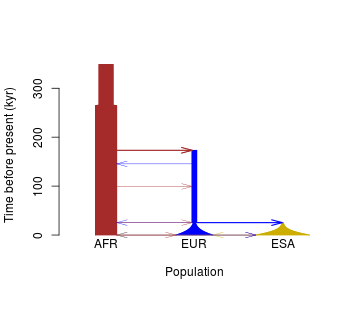[top]

###Zigzag model

cat("ms 4 1 -t 7156.0000000 -r 2000.0000 10000000 -eN 0 5 -eG 0.000582262 1318.18
-eG 0.00232905 -329.546 -eG 0.00931619 82.3865 -eG 0.0372648 -20.5966
-eG 0.149059 5.14916 -eN 0.596236 0.5 -T", file="zigzag.cmd")
par(mfrow=c(1,2), las=1)
PlotMS(input.file="zigzag.cmd", type="ms", N4=10000)
#change the time unit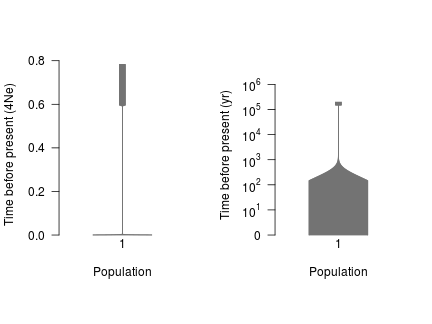[top]

###Demographic plot from msprime's script

This POPdemog package is also able to extract the demographic information from msprime by first converting an msprime script into a ms-compatible command. We use “msprime2ms.py” to translate the python simulation script into a ms-compatible command. For the ms-compatible command, users must set the 'type' parameter to be “msprime” and copy the command line to an input file or input string. Here we give an example of how to plot the demographic graph from an msprime script copied from the msprime's online documents8. Suppose msprime has been successfully installed, and the following msprime's scripts of the demographic events is saved in the file “demo1.py”.

# First we set out the maximum likelihood values of the various parameters
N_A=7300
N_B=2100
N_AF=12300
N_EU0=1000
N_AS0=510
# Times are provided in years, so we convert into generations.
generation_time=25
T_AF=220e3 / generation_time
T_B=140e3 / generation_time
T_EU_AS=21.2e3 / generation_time
# We need to work out the starting (diploid) population sizes based on
# the growth rates provided for these two populations
r_EU=0.004
r_AS=0.0055
N_EU=N_EU0 / math.exp(-r_EU * T_EU_AS)
N_AS=N_AS0 / math.exp(-r_AS * T_EU_AS)
# Migration rates during the various epochs.
m_AF_B=25e-5
m_AF_EU=3e-5
m_AF_AS=1.9e-5
m_EU_AS=9.6e-5
# Population IDs correspond to their indexes in the population
# configuration array. Therefore, we have 0=YRI, 1=CEU and 2=CHB
# initially.
population_configurations=[
msprime.PopulationConfiguration(
sample_size=0, initial_size=N_AF),
msprime.PopulationConfiguration(
sample_size=1, initial_size=N_EU, growth_rate=r_EU),
msprime.PopulationConfiguration(
sample_size=1, initial_size=N_AS, growth_rate=r_AS)
]
migration_matrix=[
[      0, m_AF_EU, m_AF_AS],
[m_AF_EU,       0, m_EU_AS],
[m_AF_AS, m_EU_AS,       0],
]

demographic_events=[
# CEU and CHB merge into B with rate changes at T_EU_AS
msprime.MassMigration(
time=T_EU_AS, source=2, destination=1, proportion=1.0),
msprime.MigrationRateChange(time=T_EU_AS, rate=0),
msprime.MigrationRateChange(
time=T_EU_AS, rate=m_AF_B, matrix_index=(0, 1)),
msprime.MigrationRateChange(
time=T_EU_AS, rate=m_AF_B, matrix_index=(1, 0)),
msprime.PopulationParametersChange(
time=T_EU_AS, initial_size=N_B, growth_rate=0, population_id=1),
# Population B merges into YRI at T_B
msprime.MassMigration(
time=T_B, source=1, destination=0, proportion=1.0),
# Size changes to N_A at T_AF
msprime.PopulationParametersChange(
time=T_AF, initial_size=N_A, population_id=0)
]


Then we use the “msprime2ms.py” to convert the demographic description into ms-compatible format:

python msprime2ms.py demo1.py population_configurations migration_matrix demographic_events


where “population_configurations” is the name of the variable for population_configurations, “migration_matrix” is the name of the variable for migration_matrix, and “demographic_events” is the name of the variable for demographic_events. Store the output to the file named “msprime.demo.cmd”

cat("
--structure 3 1 1 1
--population-size 1 0.3075
--population-size 2 0.7431335886597129
--population-growth-rate-change 0 2 160.0
--population-size 3 1.352258276948663
--population-growth-rate-change 0 3 220.0
--migration-matrix x 1.2 0.76 1.2 x 3.8400000000000003 0.76 3.8400000000000003 x
--population-split 0.0212 3 2
--population-size-change 0.0212 2 0.0525
--population-growth-rate-change 0.0212 2 0
--migration-matrix-change 0.0212 3 x 10.0 x 10.0 x x x x x
--population-split 0.14 2 1
--population-size-change 0.22 1 0.1825",
file="msprime.demo.cmd")


Then we can use the PlotMS() and PlotMig() functions to plot the demographic history.

#plot the demographic graph
par(mfrow=c(1,2),las=1)
PlotMS(input.file="msprime.demo.cmd", type="msprime", N4=4*10000,
size.scale="log", log.base=1.5, inpos=c(1,4,7), time.scale="log10year",
col.pop=c("brown", "blue", "gold3"), pops=c("AFR", "EUR", "ASIA"),
cex.lab=1, cex.axis=1, xlab="", length.arrowtip=0.1)
title("Demographic history")
NRuler("topleft", Nsize=c(1e4,1e5), Nlab=c("1e4", "1e5"), N4=40000,
size.scale="log",log.base=1.5, lwd=1, cex=0.6)
PlotMS(input.file="msprime.demo.cmd", type="msprime", N4=4*10000,
time.scale="log10year", plot.out=F, demo.out=T )->out;
PlotMig(time_pt=100, demograph_out=out$demograph_out, time.scale="year", mig_par=out$mig_par, col.pop=c("brown", "blue", "gold3"),
size.scale="topology");
legend("topleft", legend=c("AFR", "EUR", "ASIA"), col=c("brown", "blue", "gold3"), pch=20, bty="n")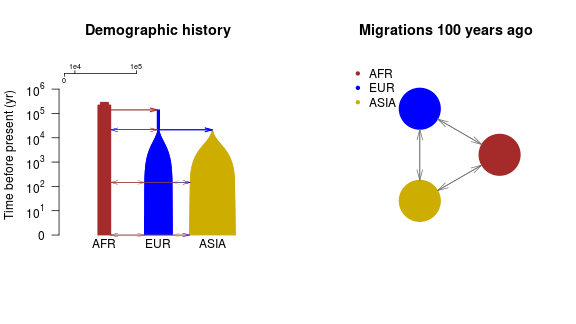[top]

###Support for SCRM simulation script

The POPdemog packagealso supports the scrm simulator. Here we make an example simulation script that changes -em 5e-04 2 1 2000 -em 6e-04 2 1 0 in the ms-style command from the file “sample.ms.cmd” to -eps 5e-04 2 1 0.8.

cat("scrm 1 1 -r 25 250001 -t 2.5 -I 4 50 50 50 60 -n 1 10 -n 2 10
-n 3 10 -n 4 10 -em 0 1 4 0.32 -em 0 4 1 0.32 -em 0 3 4 0.08
-em 0 4 3 0.08 -eps 5e-04 2 1 0.8 -ej 7e-04 2 4
-en 0.02 4 2.4 -en 0.035 1 0.77 -en 0.04 3 0.77 -en 0.1997 4 0.0125
-en 0.1998 3 0.00149253731343284 -en 0.1999 1 0.005 -ej 0.2 3 1
-em 0.1996 1 4 0 -em 0.1995 4 1 0 -em 0.1994 3 4 0 -em 0.1993 4 3 0
-en 0.3499 1 0.00117647058823529 -ej 0.35 1 4 -en 1.7 4 1.25", file="scrm.demo.cmd")
par(mfrow=c(1,2), las=1)
PlotMS(input.file="scrm.demo.cmd", type="scrm", N4=10000,
size.scale="log", log.base =50, inpos=c(3,6,1,9),
col.pop=c("blue", "coral3", "gold3", "brown"),
pops=c("European", "African American", "Asian", "African"),
title("Zoom in recent events", cex=0.8)
PlotMS(input.file="scrm.demo.cmd", type="scrm", N4=10000,
size.scale="topology", inpos=c(3,6,1,9),
col.pop=c("blue", "coral3", "gold3", "brown"),
pops=c("European", "African American", "Asian", "African"),
cex.lab=0.6, cex.axis=0.6, length.arrowtip=0.05, ylab="Time before present", m.adjust=0.05)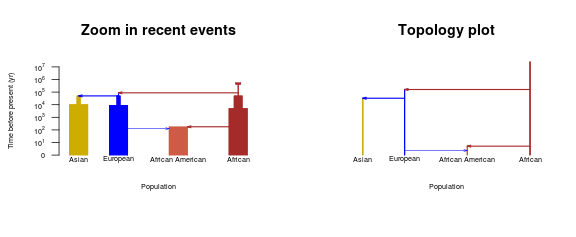[top]

##Acknowledgements Xiaowen Tian wrote the python script “msprime2ms.py”.
Brian Browning and Sharon Browning helped proof-read this tutorial and R document.

[top]

##References

: Chen, G. K., P. Marjoram, and J. D. Wall. “Fast and Flexible Simulation of DNA Sequence Data.” Genome Research 19.1 (2008): 136-42. : Hellenthal, G., and M. Stephens. “MsHOT: Modifying Hudson's Ms Simulator to Incorporate Crossover and Gene Conversion Hotspots.” Bioinformatics 23.4 (2006): 520-21. : Hudson, R. R. “Generating Samples under a Wright-Fisher Neutral Model of Genetic Variation.” Bioinformatics 18.2 (2002): 337-38. : Moorjani, Priya, et al. “A Genetic Method for Dating Ancient Genomes Provides a Direct Estimate of Human Generation Interval in the Last 45,000 Years.” Proceedings of the National Academy of Sciences, vol. 113, no. 20, Feb. 2016, pp. 5652–5657. : Shlyakhter, Ilya, Pardis C. Sabeti, and Stephen F. Schaffner. “Cosi2: An Efficient Simulator of Exact and Approximate Coalescent with Selection | Bioinformatics | Oxford Academic.” OUP Academic. Oxford University Press, 22 Aug. 2014. : Vernot, B., S. Tucci, J. Kelso, J. G. Schraiber, A. B. Wolf, R. M. Gittelman, M. Dannemann, S. Grote, R. C. Mccoy, H. Norton, L. B. Scheinfeldt, D. A. Merriwether, G. Koki, J. S. Friedlaender, J. Wakefield, S. Paabo, and J. M. Akey. “Excavating Neandertal and Denisovan DNA from the Genomes of Melanesian Individuals.” Science 352.6282 (2016): 235-39. : Jerome Kelleher, Alison M Etheridge and Gilean McVean (2016), Efficient Coalescent Simulation and Genealogical Analysis for Large Sample Sizes, PLoS Comput Biol 12(5): e1004842.

: Gutenkunst RN, Hernandez RD, Williamson SH, Bustamante CD (2009) Inferring the Joint Demographic History of Multiple Populations from Multidimensional SNP Frequency Data. PLOS Genetics 5(10): e1000695. https://doi.org/10.1371/journal.pgen.1000695 : Paul R. Staab, Sha Zhu, Dirk Metzler and Gerton Lunter. scrm: efficiently simulating long sequences using the approximated coalescent with recombination. Bioinformatics (2015) 31 (10): 1680-1682.